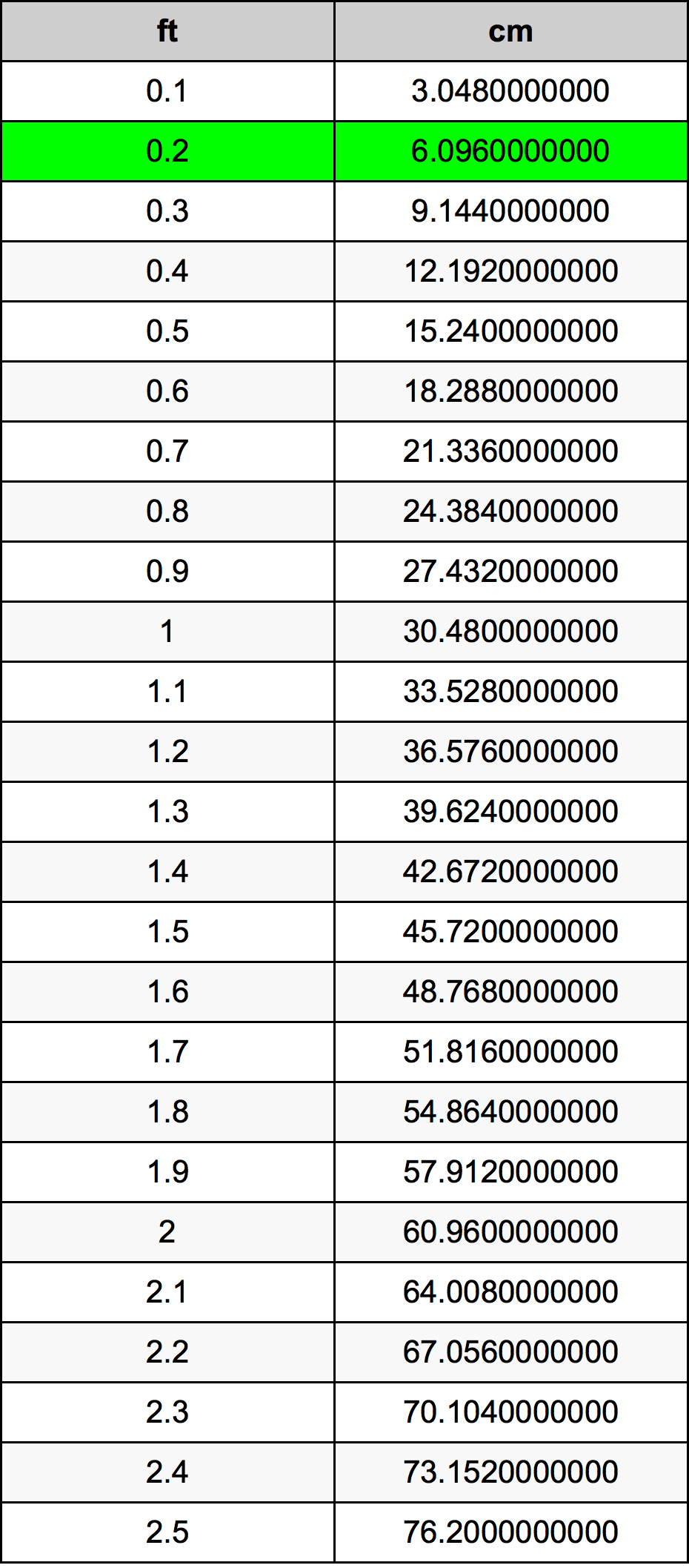Feet To Cm

# 0.2 ft to cm0.2 Feet to Centimeters

ft
=
cm

## How to convert 0.2 feet to centimeters?

 0.2 ft * 30.48 cm = 6.096 cm 1 ft
A common question is How many foot in 0.2 centimeter? And the answer is 0.0065616798 ft in 0.2 cm. Likewise the question how many centimeter in 0.2 foot has the answer of 6.096 cm in 0.2 ft.

## How much are 0.2 feet in centimeters?

0.2 feet equal 6.096 centimeters (0.2ft = 6.096cm). Converting 0.2 ft to cm is easy. Simply use our calculator above, or apply the formula to change the length 0.2 ft to cm.

## Convert 0.2 ft to common lengths

UnitLength
Nanometer60960000.0 nm
Micrometer60960.0 µm
Millimeter60.96 mm
Centimeter6.096 cm
Inch2.4 in
Foot0.2 ft
Yard0.0666666667 yd
Meter0.06096 m
Kilometer6.096e-05 km
Mile3.78788e-05 mi
Nautical mile3.29158e-05 nmi

## What is 0.2 feet in cm?

To convert 0.2 ft to cm multiply the length in feet by 30.48. The 0.2 ft in cm formula is [cm] = 0.2 * 30.48. Thus, for 0.2 feet in centimeter we get 6.096 cm.

## 0.2 Foot Conversion Table## Alternative spelling

0.2 Foot to Centimeters, 0.2 Foot in Centimeters, 0.2 Foot to Centimeter, 0.2 Foot in Centimeter, 0.2 Foot to cm, 0.2 Foot in cm, 0.2 ft to cm, 0.2 ft in cm, 0.2 Feet to cm, 0.2 Feet in cm, 0.2 ft to Centimeter, 0.2 ft in Centimeter, 0.2 Feet to Centimeter, 0.2 Feet in Centimeter